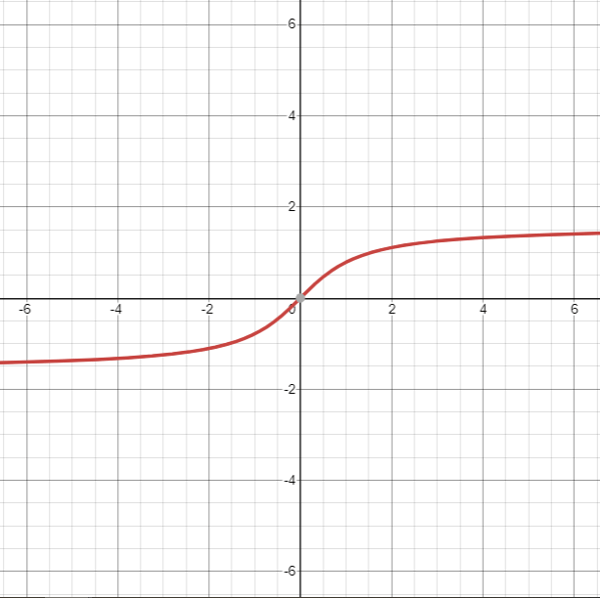Related Tags

php
math
tangent
communitycreator

# What is atan() in PHP?

harris910

The atan() functionalso called the arc tangent function returns the inverse tangent of a number. To be more specific, atan() returns the inverse tangent of a number in radians.

This means that tan(atan(x)) would be equal to x.

Figure 1 shows the mathematical representation of the atan() function.

Figure 1: Mathematical representation of inverse tangent function

To convert radians to degrees, use:

degrees = radians * ( 180.0 / M_PI )


Below is the graphical representation of the atan function.Graphical representation of atan() function

### Syntax

float atan(num)


### Parameter

This function requires a number as a parameter.

### Return value

atan() will return the inverse tangent of a number (in radians) that is sent as a parameter. The return value lies within [-pi/2,pi/2] radians.

### Example

<?php
echo("atan(0.5): ");
echo (atan(0.5));
?>
<?php
echo("atan(-0.5): ");
echo (atan(-0.5));
?>

<?php
#tan(atan(x))
echo("tan(atan(0.5)): ");
echo (tan(atan(0.5)));
?>

<?php
#applying atan() and then converting the result in radians to degrees
echo("atan(1) * (180.0 / M_PI)): ");
echo (atan(1) * (180.0 / M_PI));
echo(" Degrees")
?>



RELATED TAGS

php
math
tangent
communitycreator

CONTRIBUTOR

harris910
RELATED COURSES

View all Courses

Keep Exploring

Learn in-demand tech skills in half the time